# A.1. Molecular Orbital Theory

$$\newcommand{\vecs}{\overset { \rightharpoonup} {\mathbf{#1}} }$$ $$\newcommand{\vecd}{\overset{-\!-\!\rightharpoonup}{\vphantom{a}\smash {#1}}}$$$$\newcommand{\id}{\mathrm{id}}$$ $$\newcommand{\Span}{\mathrm{span}}$$ $$\newcommand{\kernel}{\mathrm{null}\,}$$ $$\newcommand{\range}{\mathrm{range}\,}$$ $$\newcommand{\RealPart}{\mathrm{Re}}$$ $$\newcommand{\ImaginaryPart}{\mathrm{Im}}$$ $$\newcommand{\Argument}{\mathrm{Arg}}$$ $$\newcommand{\norm}{\| #1 \|}$$ $$\newcommand{\inner}{\langle #1, #2 \rangle}$$ $$\newcommand{\Span}{\mathrm{span}}$$ $$\newcommand{\id}{\mathrm{id}}$$ $$\newcommand{\Span}{\mathrm{span}}$$ $$\newcommand{\kernel}{\mathrm{null}\,}$$ $$\newcommand{\range}{\mathrm{range}\,}$$ $$\newcommand{\RealPart}{\mathrm{Re}}$$ $$\newcommand{\ImaginaryPart}{\mathrm{Im}}$$ $$\newcommand{\Argument}{\mathrm{Arg}}$$ $$\newcommand{\norm}{\| #1 \|}$$ $$\newcommand{\inner}{\langle #1, #2 \rangle}$$ $$\newcommand{\Span}{\mathrm{span}}$$$$\newcommand{\AA}{\unicode[.8,0]{x212B}}$$

The Molecular Orbital Theory, initially developed by Robert S. Mullikan, incorporates the wave like characteristics of electrons in describing bonding behavior. In Molecular Orbital Theory, the bonding between atoms is described as a combination of their atomic orbitals. While the Valence Bond Theory and Lewis Structures sufficiently explain simple models, the Molecular Orbital Theory provides answers to more complex questions. In the Molecular Orbital Theory, the electrons are delocalized. Electrons are considered delocalized when they are not assigned to a particular atom or bond (as in the case with Lewis Structures). Instead, the electrons are “smeared out” across the molecule. The Molecular Orbital Theory allows one to predict the distribution of electrons in a molecule which in turn can help predict molecular properties such as shape, magnetism, and Bond Order.

## Introduction

Atoms form bonds by sharing electrons. Atoms can share two, four, or six electrons, forming single, double, and triple bonds respectively. Although it is impossible to determine the exact position of an electron, it is possible to calculate the probability that one will find the electron at any point around the nucleus using the Schrödinger Equation. This equation can help predict and determine the energy and spatial distribution of the electron, as well as the shape of each orbital. The figure below shows the first five solutions to the equation in a three dimensional space for a one electron atom. The colors show the phase of the function. In this diagram, blue stands for negative and red stands for positive. Note, however, that the 2s orbital has 2 phases, one of which is not visible because it is inside the other.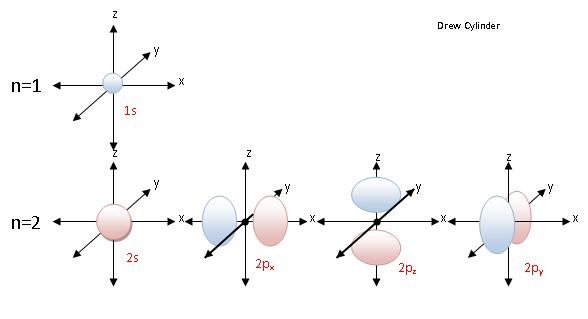Figure 1: Cartoons of the volume occupied by electrons in the 1s, 2s, and 2p hydrogen-like orbitals.

## Principles of Molecular Orbital Theory

In molecules, atomic orbitals combine to form molecular orbitals which surround the molecule. Similar to atomic orbitals, molecular orbitals are wave functions giving the probability of finding an electron in certain regions of a molecule. Each molecular orbital can only have 2 electrons, each with an opposite spin. Each molecular orbital can only have 2 electrons, each with an opposite spin. Once you have the molecular orbitals and their energy ordering the ground state configuration is found by applying the Pauli principle, the aufbau principle and Hund's rule just as with atoms.

The principles to apply when forming pictorial molecular orbitals from atomic orbitals are summarized in the table below:

Principle Details/Examples

Total number of molecular orbitals is equal to the total number of atomic orbitals used to make them.

The molecule H2 is composed of two H atoms. Both H atoms have a 1s orbital, so when bonded together, there are therefore two molecular orbitals.

Bonding molecular orbitals are lower energy than the atomic orbitals from which they were formed.

Antibonding molecular orbitals are higher energy than the atomic orbitals from which they were formed.

Electrons in bonding molecular orbitals help stabilize a system of atoms since less energy is associated with bonded atoms as opposed to a system of unbound atoms. Bonding orbitals are formed by in-phase combinations of atomic orbitals and increase the electron density between the atoms (see figure 2 below)

Electrons in antibonding molecular orbitals cause a system to be destabilized since more energy is associated with bonded atoms than that of a system of unbound atoms. Antibonding orbitals are formed by out-of-phase combinations of atomic orbitals and decrease the electron density between atoms (see figure 2 below).

Following both the Pauli exclusion principle and Hund's rule, electrons fill in orbitals of increasing energy.

Electrons fill orbitals with the lowest energy first. No more than 2 electrons can occupy 1 molecular orbital at a time. Furthermore, all orbitals at an energy level must be filled with one electron before they can be paired. (see figure 3 below)

Molecular orbitals are best formed when composed of Atomic orbitals of like energies.

When Li2 forms the two lowest energy orbitals are the pair of bonding and antibonding orbitals formed from the two possible combinations of the 1s on each atom. The 2s orbitals combine primarily with each other to form another pair of bonding and antibonding orbitals at a higher energy.

Figure 2 (below) shows how bonding and antibonding σ orbitals can be formed by combining s orbitals in-phase (bonding, bottom) and out-of-phase (antibonding, top). If the atomic orbitals are combined with the same phase they interfere constructively and a bonding orbital is formed. Bonding molecular orbitals have lower energy than the atomic orbitals from which they were formed. The lowering of the energy is attributed to the increase in shielding of the nuclear repulsion because of the increase in electron density between the nuclei. If the atomic orbitals are combined with different phases, they interfere destructively and an antibonding molecular orbital is formed. Antibonding molecular orbitals have a higher energy than the atomic orbitals from which they were formed. The higher energy is attributed to the reduced shielding of the nuclear repulsion because of the lower electron probability density between the nuclei.Figure 2: Combining hydrogen-like s orbitals to generate bonding (bottom) and antibonding (top) orbitals. The dark dot represents the location of the nucleus. Note the decrease in electron density between the nuclei in the antibonding orbital.

## $$\sigma$$ Bonds

Molecular orbitals that are symmetrical about the axis of the bond are called sigma molecular orbitals, often abbreviated by the Greek letter $$\sigma$$. Figure 2 shows the 1s orbitals of 2 Hydrogen atoms forming sigma orbitals. There are two types of sigma orbitals formed, antibonding sigma orbitals (abbreviated $$\sigma^*$$), and bonding sigma orbitals (abbreviated $$\sigma$$). In sigma bonding orbitals, the in phase atomic orbitals overlap causing an increase in electron density along the bond axis. Where the atomic orbitals overlap, there is an increase in electron density and therefore an increase in the intensity of the negative charge. This increase in negative charge causes the nuclei to be drawn closer together. In sigma antibonding orbitals ($$\sigma^*$$), the out of phase 1s orbitals interfere destructively which results in a low electron density between the nuclei as seen on the top of the diagram.

The diagram below (figure 3) is a representation of the energy levels of the bonding and antibonding orbitals formed in the hydrogen molecule. Two molecular orbitals were formed: one antibonding ($$\sigma^*$$) and one bonding ($$\sigma$$).The two electrons in the hydrogen molecule have antiparallel spins. Notice that the $$\sigma^*$$ orbital is empty and has a higher energy than the $$\sigma$$ orbital.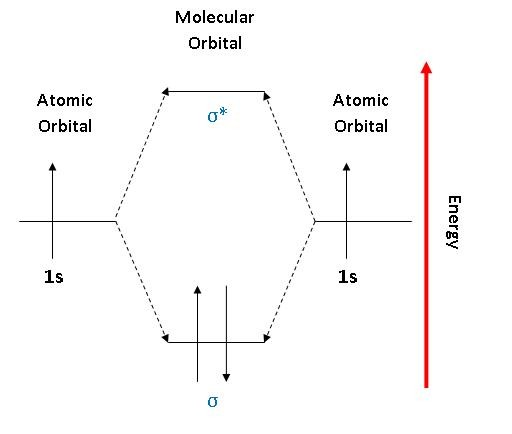Figure 3: An MO energy level diagram for H2. The up and down arrows represent electrons that are spin up or spin down.

Sigma bonding orbitals and antibonding orbitals can also be formed between p orbitals (figure 4). Notice that the orbitals have to be in phase in order to form bonding orbitals. Sigma molecular orbitals formed by p orbitals are often differentiated from other types of sigma orbitals by adding the subscript p below it. So the antibonding orbital shown in the diagram below would be σ*p.Figure 4: The formation of a σ bonding and antibonding orbital using p-orbitals.

## $$\pi$$ Bonds

The $$\pi$$ bonding is a side to side overlap of orbitals, which then causes there to be no electron density along the axis, but there is density above and below the axis. The diagram below (figure 5) shows a $$\pi$$ antibonding molecular orbital and a $$\pi$$ bonding molecular orbital.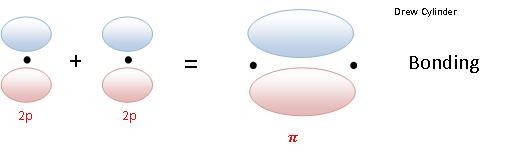Figure 5: The side on overlap of p orbitals to form pi bonding and antibonding orbitals. Note that there is a second set of p orbitals sticking in and out of the image that can combine in the same way. (see cartoons immediately below)

2py OrbitalsThe two 2py atomic orbitals overlap in parallel to form two $$\pi$$ molecular orbitals which are asymmetrical about the axis of the bond.

2pz orbitalsThe two 2pz orbitals overlap to create another pair of pi 2p and pi *2p molecular orbitals. The 2pz-2pz overlap is similar to the 2py-2py overlap because it is just the orbitals of the 2pz rotated 90 degrees about the axis. The new molecular orbitals have the same potential energies as those from the 2py-2py overlap.

In summary the three pairs of p orbitals can combine to form one set of \sigma\ orbitals and two sets of \pi\ orbitals.

## Drawing Molecular Orbital Diagrams

1. Determine how many valence electrons you have on each atom (you can ignore the core electrons as core orbitals contribute little to molecular orbitals). This gives you the total number of electrons you will have to distribute among the molecular orbitals you form. For example consider B2 (each atom has an electron configuration of [He]2s22p), which has a total of 6 valence electrons.
2. Draw a cartoon energy level diagram with lines for the valence atomic energy levels (orbitals) of each atom. Put one atom's levels on the left and one on the right. Include the electrons. Leave space in the middle for your molecular energy levels (orbitals). It can help to include cartoons of the atomic orbitals as well. Shown for diboron immediately below.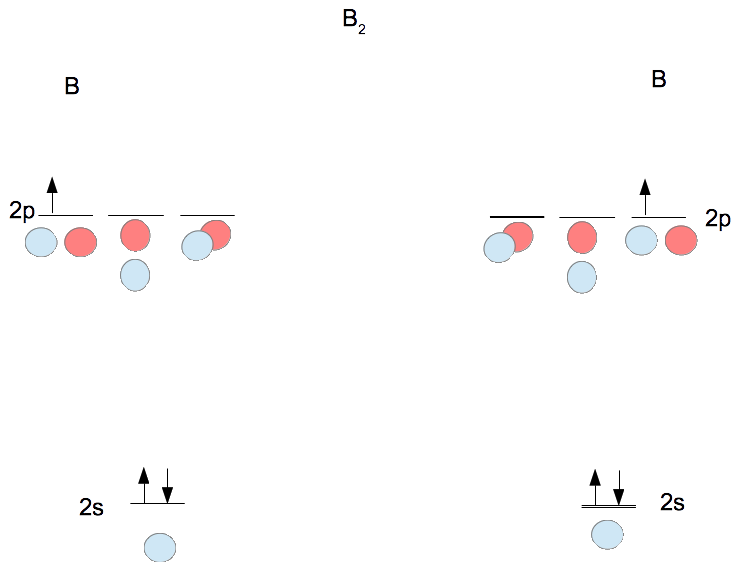3. Combine each pair of orbitals of similar energy in-phase and out-of-phase to create molecular orbitals as shown for diboron in the figure following step 4.
4. Move the electrons from the atoms into the molecule to determine the molecular electron configuration as shown immediately below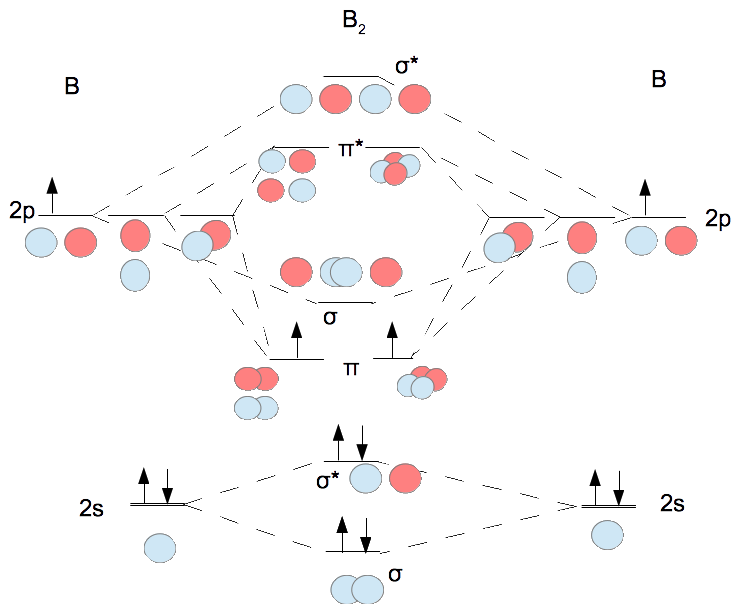Note that the σ and π orbitals formed from the p's are not always in the order π energy less than σ energy. For first row diatomics the ordering shown above is valid for Z ≤ 7. Thus for oxygen and fluorine the σ is below the π orbitals.

## Bond Orders and Stability of Molecules

Bond Order indicates the strength of the bond with the greater the bond order, the stronger the bond.

$\text{Bond Order}= \dfrac{1}{2} \left(a-b\right)$

where

• $$a$$ is the number of electrons in bonding molecular orbitals and
• $$b$$ is the number of electrons in antibondng molecular orbitals.

If the bond order is zero, then no bonds are produced and the molecule is not stable (for example $$He_2$$). If the Bond Order is 1, then it is a single covalent bond. The higher the Bond Order, the more stable the molecule is. An advantage of Molecular Orbital Theory when it comes to Bond Order is that it can more accurately describe partial bonds (for example in H2+, where the Bond Order=1/2), than Lewis Structures.

## References

1. Petrucci, RH et al. (2007). General Chemistry: Principles and Modern Applications. New Jersey: Pearson Prentice Hall.
2. Dingrando, Laurel, Kathleen Tallman, Nicholas Hainen, and Cheryl Wistrom. Chemistry. Glencoe/McGraw-Hill School Pub Co, 2004.
3. Kotz, John C., Paul M. Treichel, and Gabriela C. Weaver. "Bonding and Molecular Structure:Orbital Hybridization and Molecular Orbitals." Chemistry & Chemical Reactivity. Belmont, CA: Thomson Brooks/Cole, 2006. 457-66. Print.

A.1. Molecular Orbital Theory is shared under a CC BY-NC-SA 4.0 license and was authored, remixed, and/or curated by LibreTexts.